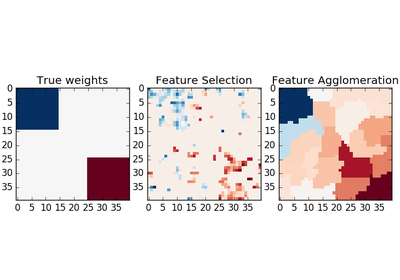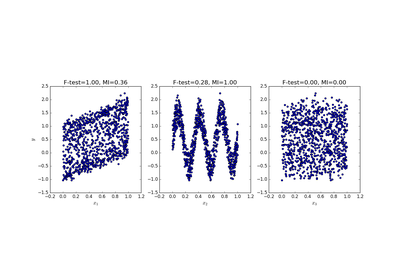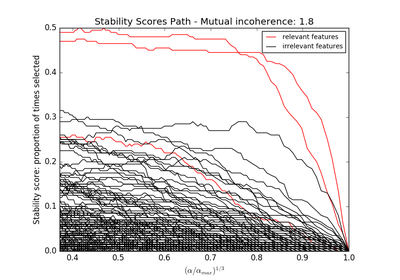# `sklearn.feature_selection`.f_regression¶

`sklearn.feature_selection.``f_regression`(X, y, center=True)[source]

Univariate linear regression tests.

Quick linear model for testing the effect of a single regressor, sequentially for many regressors.

This is done in 2 steps:

1. The cross correlation between each regressor and the target is computed, that is, ((X[:, i] - mean(X[:, i])) * (y - mean_y)) / (std(X[:, i]) * std(y)).
2. It is converted to an F score then to a p-value.

Read more in the User Guide.

Parameters: X : {array-like, sparse matrix} shape = (n_samples, n_features) The set of regressors that will be tested sequentially. y : array of shape(n_samples). The data matrix center : True, bool, If true, X and y will be centered. F : array, shape=(n_features,) F values of features. pval : array, shape=(n_features,) p-values of F-scores.

`f_classif`
ANOVA F-value between label/feature for classification tasks.
`chi2`
Chi-squared stats of non-negative features for classification tasks.

## Examples using `sklearn.feature_selection.f_regression`¶Feature agglomeration vs. univariate selectionPipeline Anova SVMComparison of F-test and mutual informationSparse recovery: feature selection for sparse linear models